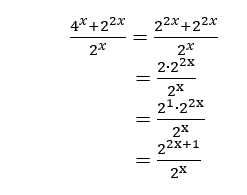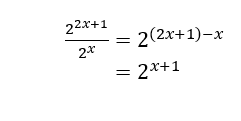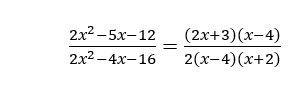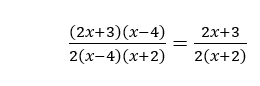# Algebra Practice Test 2

A 6
B 2+2x
C 2×2x
D 2x+1

A (x-6)/2(x-2)
B (x-6)/2(x+2)
C (2x+3)/2(x-2)
D (2x+3)/2(x+2)

A f(x)=x2-4x-5
B f(x)=x2-4x+13
C f(x)=x2-6ix-5
D f(x)=x2-6ix+13

A x<-4
B -4
C 0
D x>3/2

A 2 seconds
B 5/2 seconds
C 3 seconds
D 4 seconds

A x=-8
B x=0
C x=4
D x=8

A 6
B 20/3
C 24
D 72

A 1
B (x-3)/(x+3)
C (x2+2)/(x-3)
D (x2+2)/(x+3)

A tan ? = 1/13
B tan ? = 13
C tan ? = 5/12
D tan ? = 12/5

##### 10. Which expression is equivalent to 6√x+10x?

A 2(3x-1+5x)
B 2(3x1/2+5x)
C 2x(3x-1+5)
D 2x(3x1/2+5)

###### 1. D

You can solve this problem either (1) by simplifying the numerator and denominator separately and then simplifying the result or (2) by using the distributive property. For this problem, we will use the first method.
First rewrite 4x as an exponent of 2 using the property, (bx )y=bxy.

4x=(22 )x=22x

Then use this to simplify the numerator with the property, bx x y = bx+y.Finally, simplify the result using bx / by = bx-y###### 2. D

To simplify the expression, first factor the numerator and the denominator. By the trial-and-error method, the numerator can be factored into two binomials as follows.

2x2 – 5x – 12 = (2x + 3)(x -4 )

For the denominator, factor out the common factor, which is 2.

2x2 – 4x – 16
=2(x2 – 2x -8)
=2(x-4)(x+2)

Thus, the factored form of the expression isNotice that there is a common factor, (x – 4), which is in both the numerator and the denominator. Therefore, you can further simplify the expression by cancelling it out.###### 3. B

The roots of a quadratic function f(x) are the values of x for which f(x)=0. A quadratic function written in the form f(x)=(x-a)(x-b) has roots at x=a and x=b. Therefore, to find f(x), substitute 2-3i and 2+3i for a and b into this equation and simplify the result. Note that (2-3i)(2+3i)=4-9i2=13.

f(x)=(x-a)(x-b)
=[x-(2-3i)][x-(2+3i)]
=x2-(2-3i)x-(2+3i)x+(2-3i)(2+3i)
=x2-2x+3ix-2x-3ix+13
=x2-4x+13

###### 4. A and C

To solve, first factor the polynomial. Notice that the greatest common factor (GCF) of the terms is 2x. Factor this expression out and then use trial-and-error to factor the resulting trinomial.

4x3+10x2-24x
=2x(2x2+5x-12)
=2x(2x-3)(x+4)

Solving for 0, we find that the roots of the polynomial are x=0, x=3/2, and x=-4.

These values divide the number line into four intervals. Choose a test number from each interval and determine whether the product is positive or negative. For this problem, we will use -5, -1, 1, and 2 as test numbers. Substitute these values into the original polynomial.

x=-5:
4(-5)3+10(-5)2-24(-5)
=-375+250+120
=-5

x=-1:
4(-1)3+10(-1)2-24(-1)
=-4+10+24
=30

x=1:
4(1)3+10(1)2-24(1)
=4+10-24
=-10

x=2:
4(2)3+10(2)2-24(2)
=32+40-48
=24

Thus, the given inequality, 4x3+10x2-24x<0, is satisfied by numbers less than -5 and numbers between 0 and 3/2.

###### 5. D

The baseball will hit the ground when its height is zero. In mathematical notation, this will happen when h(t)=0. Therefore, we need to set the given function equal to zero.

h(t)=0
-16t2+46t+6=0

Now solve the resulting equation. Factor the left side and use the zero-product property to solve for t.

-2(8t2-23t-3)=0
-1(8t+1)(t-3)=0
t=-1/8 t=3

The answer only makes sense when t is positive, so we can discard the negative value. Thus, the calculator will hit the ground 3 seconds after it is thrown.

###### 6. C

nly: The logarithm of a number is the exponent that the base must to be raised to in order to get that number. For example, since 23=8, it is also true that log_2?8=3. Thus, the given equation log_2?(8x-x2 )=4 implies that

8x-x2=24

Simplify this equation and solve for x.

8x-x2=16
0=x2-8x+16
0=(x-4)2
x=4

Thus, the solution is x=4. Check this value on your own by substituting it into the original equation to make sure that the result is a true statement.

###### 7. C

The average rate of change of a function f between x=a and x=b can be computed with the formula

Average rate of change=(f(b)-f(a))/(b-a)
To use this formula, first calculate f(1) and f(4).

Then use these values to calculate the average rate of change.

Average rate of change=(f(4)-f(1))/(4-1)

###### 8. D

To simplify the expression, first factor the numerator and the denominator. The numerator can be factored by grouping as follows.

x3-3x2+2x-6
=x2 (x-3)+2(x-3)
=(x2+2)(x-3)

For the denominator, factor using the difference of squares formula, a2-b2=(a+b)(a-b).

x2-9=(x+3)(x-3)

Thus, the factored form of the expression is

(x3-3x2+2x-6)/(x2-9)
=(x2+2)(x-3)/(x+3)(x-3)

Notice that there is a common factor, (x-3), which is in both the numerator and the denominator. Therefore, you can further simplify the expression by cancelling it out.

(x2+2)(x-3)/(x+3)(x-3)
=(x2+2)/(x+3)

###### 9. C

Use a unit circle to model the value of cosine. In a right triangle, the cosine function is cos?=adjacent/hypotenuse. Using the Pythagorean Theorem, we find that the length of the second leg is

b=√(c2-a2 )
=√(12-(12/13)2 )
=√(25/169)
=5/13

Since the tangent function is tan?=opposite/adjacent, the value of tan? is tan?=√(5/13)/(12/13)=√5/12 In Quadrant I, the values of cosine and tangent are both positive. Therefore, tan?=5/12.

###### 10. B

All of the choices involve two transformations of the given expression: factoring out either 2 or 2x and changing the radical to an exponent. First factor out the greatest common factor (GCF) of the terms. In this case, the GCF is 2.

6√x+10x
=2(3√x+5x)

In addition, the square root of x is equal to x raised to the 1/2 power.

2(3√x+5x)
=2(3x1/2+5x)